Miscellaneous

Chapter 10 Class 11 Straight Lines (Term 1)
Serial order wise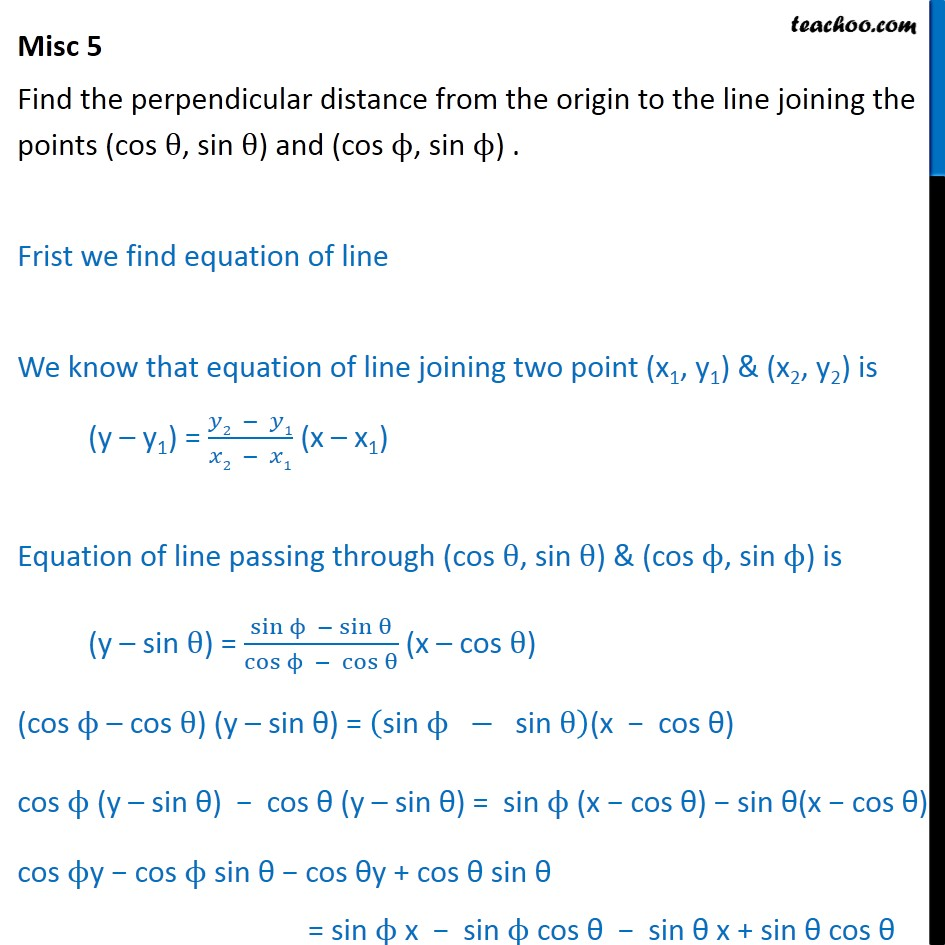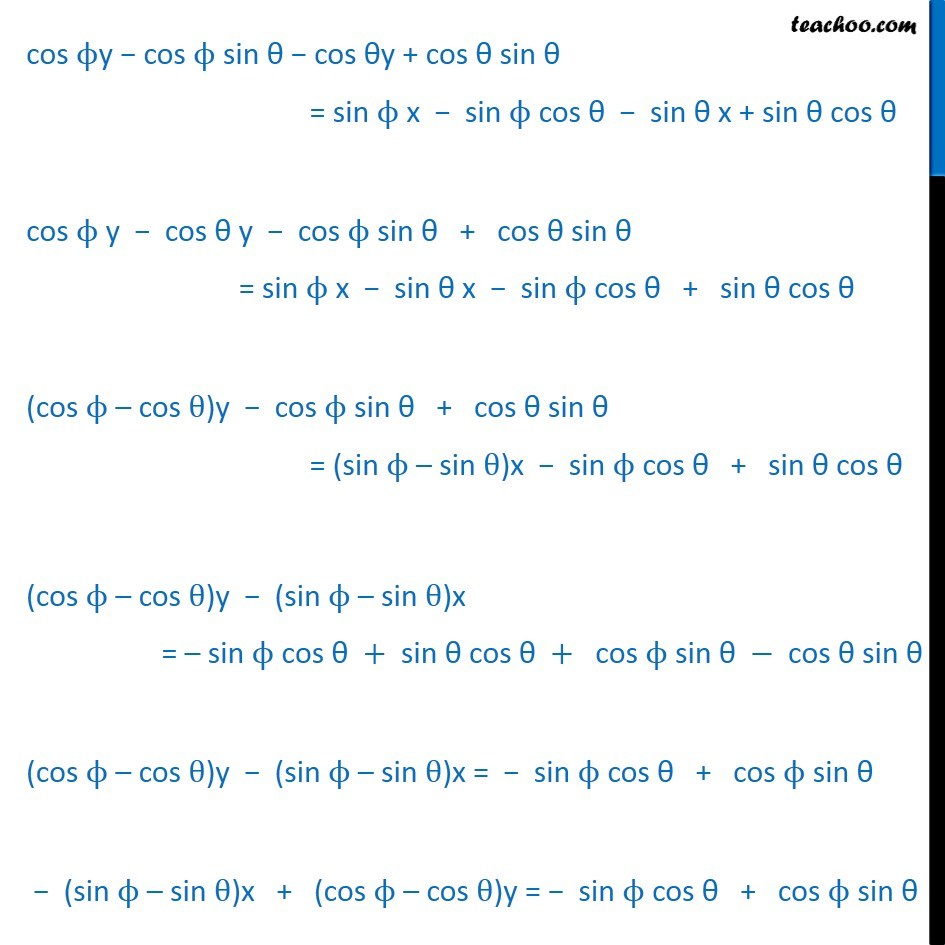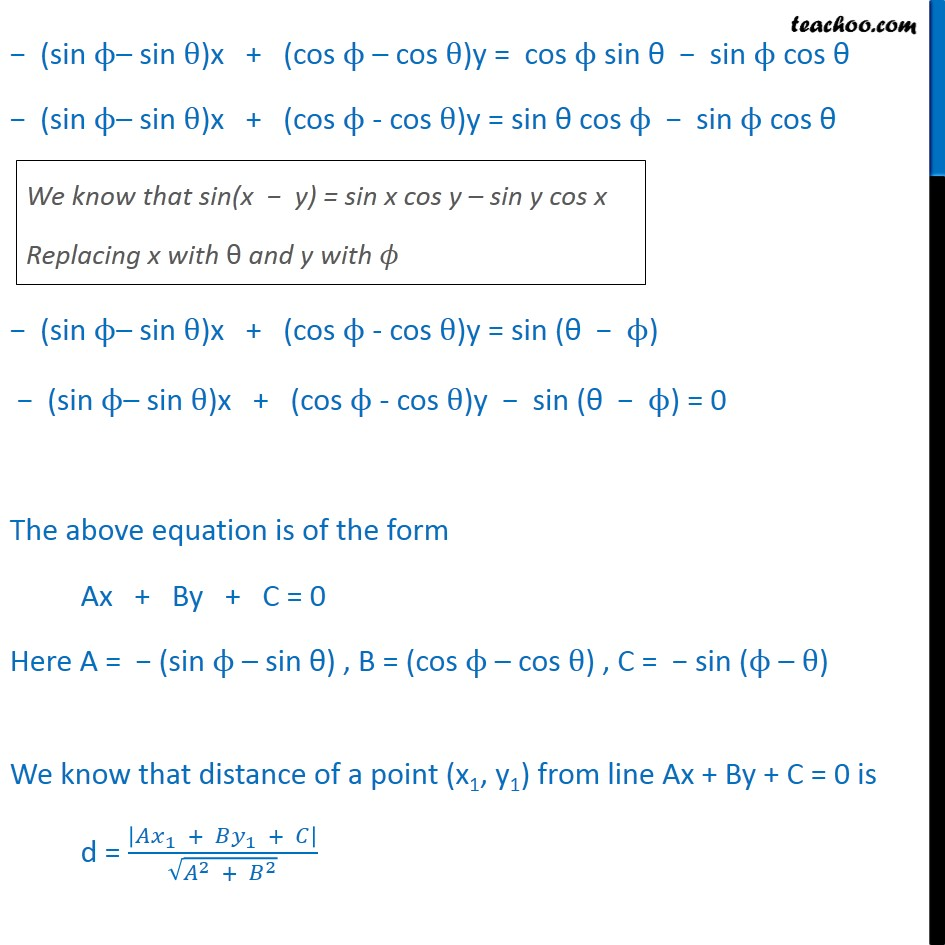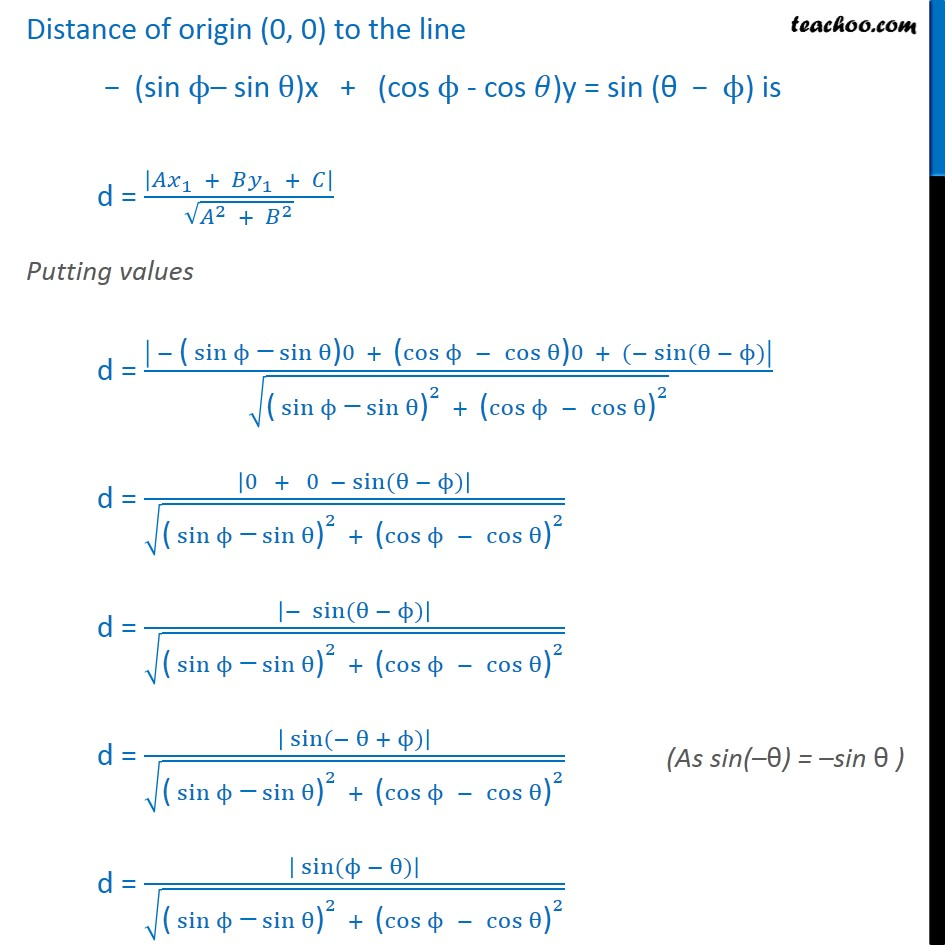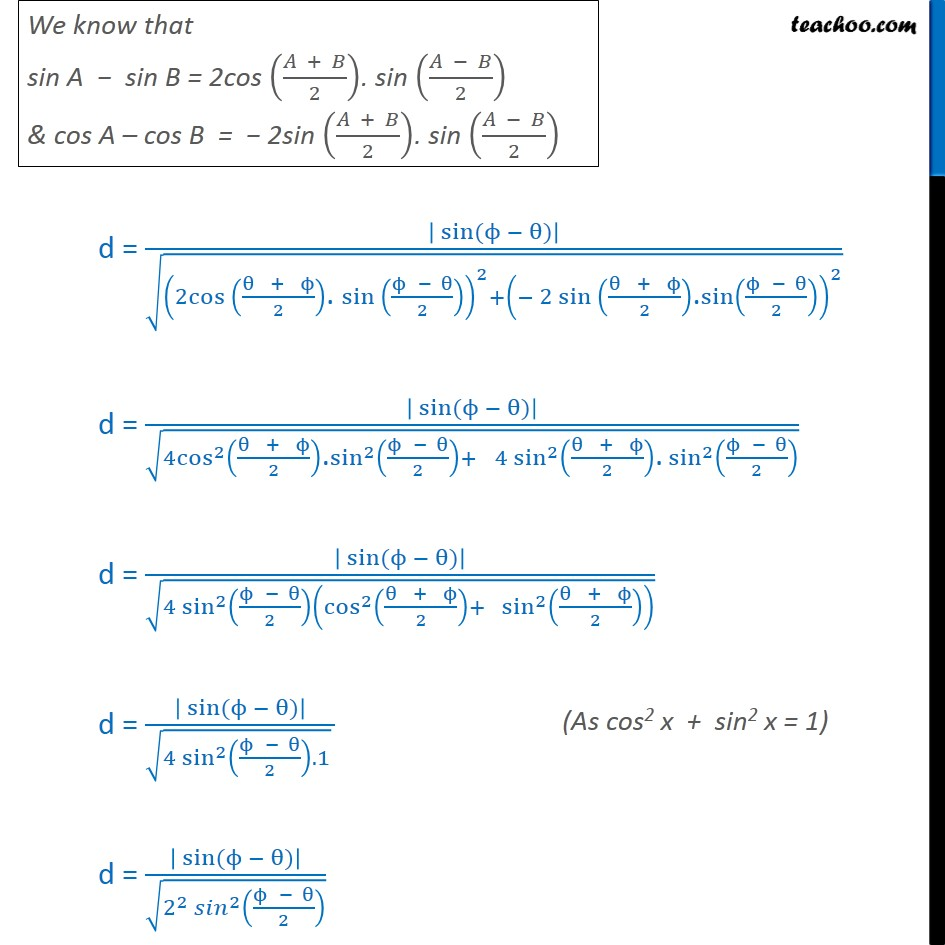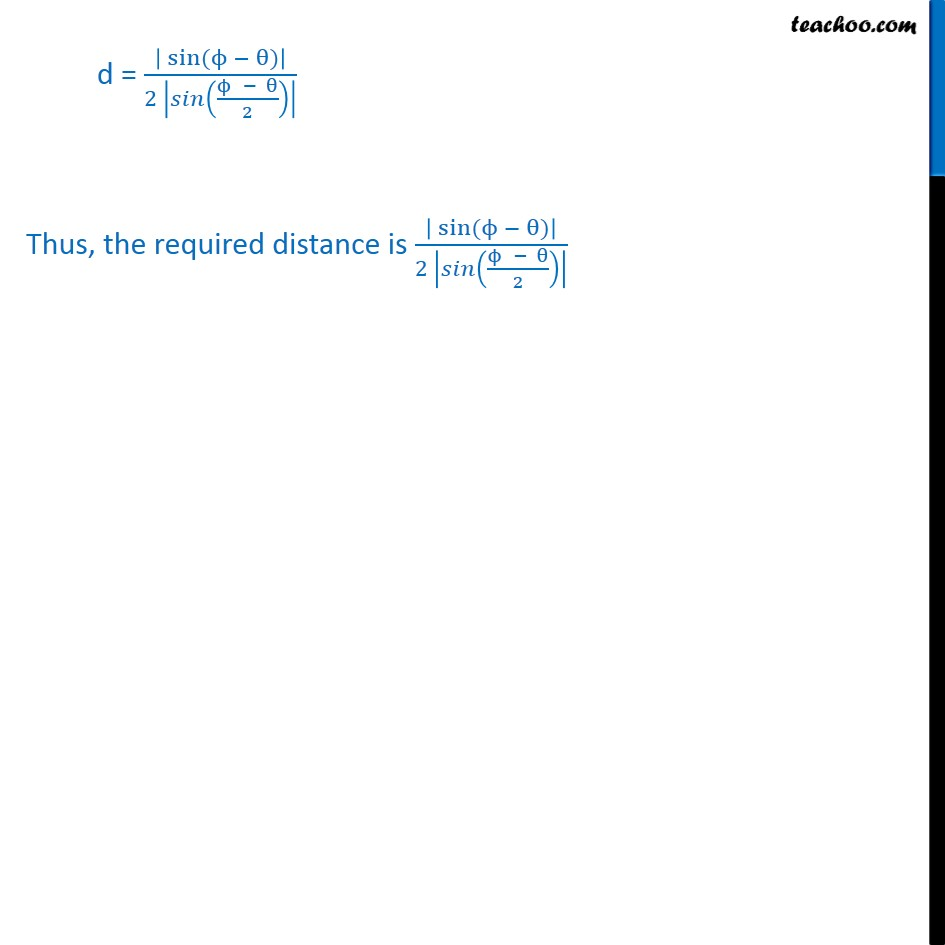### Transcript

Misc 5 Find the perpendicular distance from the origin to the line joining the points (cos , sin ) and (cos , sin ) . Frist we find equation of line We know that equation of line joining two point (x1, y1) & (x2, y2) is (y y1) = ( 2 1)/( 2 1) (x x1) Equation of line passing through (cos , sin ) & (cos , sin ) is (y sin ) = (sin sin )/(cos cos ) (x cos ) (cos cos ) (y sin ) = ("sin " " " " sin " )(x cos ) cos (y sin ) cos (y sin ) = "sin " (x cos ) sin (x cos ) cos y cos sin cos y + cos sin = sin x sin cos sin x + sin cos cos y cos sin cos y + cos sin = sin x sin cos sin x + sin cos cos y cos y cos sin + cos sin = sin x sin x sin cos + sin cos (cos cos )y cos sin + cos sin = (sin sin )x sin cos + sin cos (cos cos )y (sin sin )x = "sin " " cos "+" sin cos "+ " cos " " sin " "cos sin " (cos cos )y (sin sin )x = sin cos + cos sin (sin sin )x + (cos cos )y = sin cos + cos sin (sin sin )x + (cos cos )y = cos sin sin cos (sin sin )x + (cos - cos )y = sin cos sin cos (sin sin )x + (cos - cos )y = sin ( ) (sin sin )x + (cos - cos )y sin ( ) = 0 The above equation is of the form Ax + By + C = 0 Here A = (sin sin ) , B = (cos cos ) , C = sin ( ) We know that distance of a point (x1, y1) from line Ax + By + C = 0 is d = | _1 + _1 + |/ ( ^2 + ^2 ) Distance of origin (0, 0) to the line (sin sin )x + (cos - cos )y = sin ( ) is d = | _1 + _1 + |/ ( ^2 + ^2 ) Putting values d = | "(" sin " " sin ")" 0 + "(" cos " " " " cos ")" 0 + ( sin ( )|/ ( "(" sin " " sin ")" ^2 + "(" cos " " " " cos ")" ^2 ) d = |0 + 0 sin ( )|/ ( "(" sin " " sin ")" ^2 + "(" cos " " " " cos ")" ^2 ) d = | sin ( )|/ ( "(" sin " " sin ")" ^2 + "(" cos " " " " cos ")" ^2 ) d = | sin ( + )|/ ( "(" sin " " sin ")" ^2 + "(" cos " " " " cos ")" ^2 ) d = | sin ( )|/ ( "(" sin " " sin ")" ^2 + "(" cos " " " " cos ")" ^2 ) d = | sin ( )|/ ((2cos (( " " + " " )/2)". " sin (( " " )/2))^2+( 2 sin (( " " + " " )/2)"." sin(( " " )/2))^2 ) d = | sin ( )|/ (4cos^2 (( " " + " " )/2)"." sin^2 (( " " )/2)+ " " 4 sin ^2 (( " " + " " )/2)"." sin^2 (( " " )/2) ) d = | sin ( )|/ (4 sin ^2 (( " " )/2)(cos^2 (( " " + " " )/2)+ sin ^2 (( " " + " " )/2)) ) d = | sin ( )|/( (4 sin ^2 (( " " )/2).1) ) d = | sin ( )|/ (2^2 ^2 (( " " )/2) ) d = | sin ( )|/(2 | (( " " )/2)| ) Thus, the required distance is | sin ( )|/(2 | (( " " )/2)| )